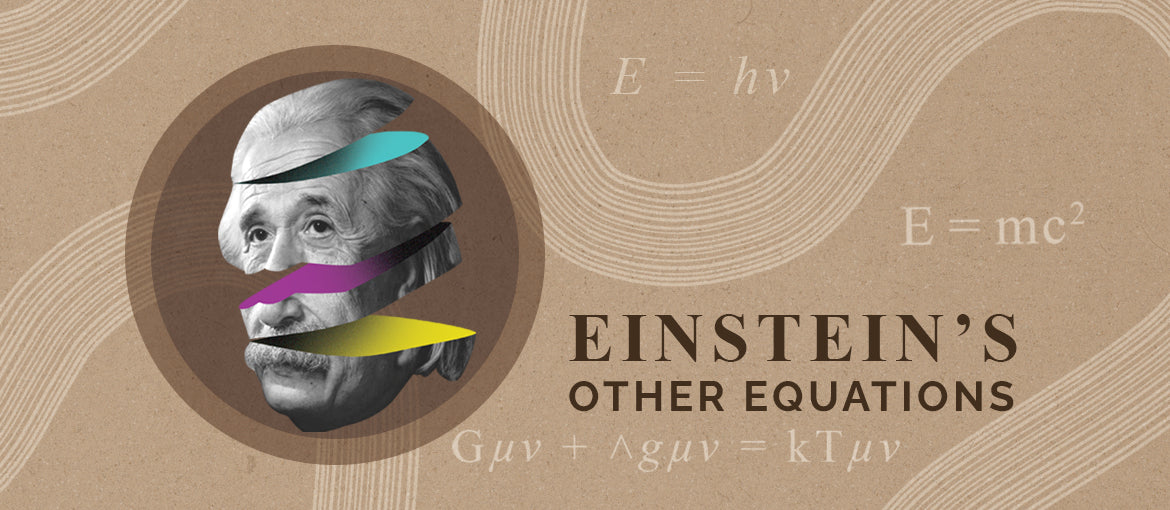Einstein’s Other Equations

Albert Einstein is the most famous physicist, and arguably the most famous scientific figure of all time. He published hundreds of papers and won countless prizes and accolades, most notably a Nobel Prize and ‘TIME person of the century’ in 1999. From special relativity, gravitational waves to the famous

𝐸=𝑚𝑐2

mass-energy equivalence, Einstein’s work is well known and celebrated across the globe. In a career that spanned over 50 years and two continents, there is clearly far more to his work than most would know: here are three of his lesser known but massively influential concepts and formula.

Einstein’s Relation

𝐸= ℎ𝑣

Also known as the Planck-Einstein Relation or Planck Equation, this formula describes the nature of natural light, quantifying the energy 𝐸 of a photon of light in relation to its frequency 𝑣. Einstein developed the theory alongside fellow German theoretical physicist Max Planck, after whom ‘Planck’s constant, ℎ’ is named, and is integral to the understanding of the photoelectric effect when studying quantum mechanics.

Bose-Einstein Condensate

Satyendra Nath Bose was an Indian born theoretical physicist who developed theory for Quantum statistics of photons. He compiled his findings into a paper which he sent across the globe to Einstein. Einstein was instantly impressed and began to devote his studies to the same area, leading to the prediction of a state of matter that was rightly named the Bose-Einstein Condensate. Also described as the fifth state of matter, it occurs when a gas of boson at low temperatures is cooled to temperatures close to absolute zero, at which point a fraction of these bosons will demonstrate quantum mechanical phenomena such as wavefunction interference at a macroscopic level.

Einstein field equations

𝐺𝜇𝜈 + Λ𝑔𝜇𝜈 = 𝜅𝑇𝜇𝜈

First published in 1915, Einstein’s field equations describe the distribution of matter with regard to the geometry of space time. The publication controversially included this term ‘Λ′, the cosmological constant, which works under the assumption that the universe is neither expanding nor contracting, something that was not supported by the observations of Edward Hubble at the time. He later abandoned it for other theories once admitting ‘that the introduction of the cosmological term was the biggest blunder of his life’. (Gamow)